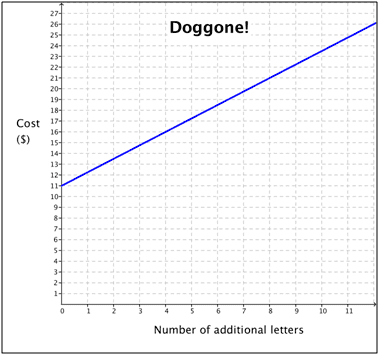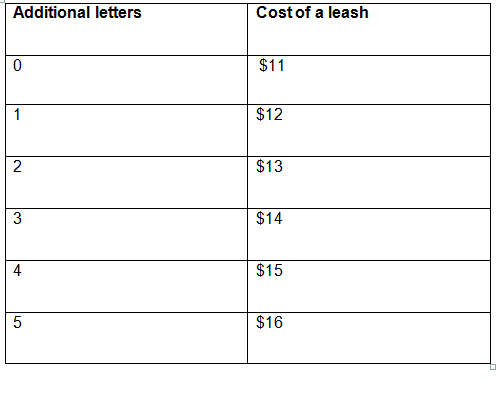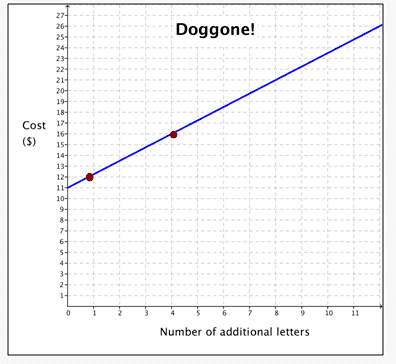# Writing equations and calculating cost based on a graph

• Kirito123
In summary, the Doggone Leash Company charges an additional fee for each letter after the first 10. The cost of a leash with no additional letters is $11. #### Kirito123 ## Homework Statement 12. The Doggone Leash Company offers personalized dog accessories. The dog’s name is printed on items such as leashes and collars. A basic fee is charged for the first 10 letters of the dog’s name, and an additional charge applies to each letter after that. The graph shows the relationship between the total cost of the service and the number of additional letters in the dog’s name.a. Write an equation that models this relationship and define the variables that you use. b.What is the cost of each additional letter? Explain how you know. c.What is the cost of a leash with no additional letters? Explain how you know. d. Create a table of values that shows the cost of a leash for 0 to 5 extra letters. 2. Homework Equations y=mx+b ## The Attempt at a Solution so here are my answers, are they correct?[/B] a. Write an equation that models this relationship and define the variables that you use. In the equation y = mx + b, y represents the total cost of personalized dog accessories, m represents the basic fee, x represents the number of 10 letters, and b represents the additional fee. According to the graph the basic fee is$11. I also noticed every time an additional letter is added the cost increases by $1, meaning the additional fee is$1.
An equation that models this relationship is:

y = 11x + 1

b. What is the cost of each additional letter? Explain how you know.
By looking at the graph I can tell that the cost is increasing by $1 for each additional letter added on. This indicates that the cost of an additional letter is$1.

c. What is the cost of a leash with no additional letters? Explain how you know.
Since there are no additional letters the amount of letter purchased is 10. The basic fee for 10 letters is $11. Therefore, the cost of a leash is$11.

d. Create a table of values that shows the cost of a leash for 0 to 5 extra letters.The equation of the line is wrong. According to the graph, when x = 0, you don't have additional letters, it costs 11$. When I plug x = 0 in your equation, it would cost 1$.

Do you know what the meaning is of m? And how m is defined?

by m you mean slope? As in the formula y=mx+b?

Yes

wait so the m value i got is wrong? would it perhaps be y =10x+1?

Oh I get what you mean when you do 11 x 0 you get 0 then add 1 so the total would be $1. So how would i fix the equation? Wait could i do y= 1x +11? If you subisitiue x for 0 it would work Kirito123 said: Oh I get what you mean when you do 11 x 0 you get 0 then add 1 so the total would be$1. So how would i fix the equation? Wait could i do y= 1x +11? If you substitute x for 0 it would work
That gives the correct y-intercept.

However, it looks like you are just guessing at the slope.

Last edited:
SammyS said:
That give the correct y-intercept.

However, it looks like you are just guessing at the slope.

Yes, he seems to be guessing the slope. I repeat my question for the OP. Do you know what the m in y = mx + b represent? And how it is mathematically defined?

Kirito123 said:

## Homework Statement

12. The Doggone Leash Company offers personalized dog accessories. The dog’s name is printed on items such as leashes and collars. A basic fee is charged for the first 10 letters of the dog’s name, and an additional charge applies to each letter after that. The graph shows the relationship between the total cost of the service and the number of additional letters in the dog’s name.
View attachment 99421

a. Write an equation that models this relationship and define the variables that you use.
b.What is the cost of each additional letter? Explain how you know.
c.What is the cost of a leash with no additional letters? Explain how you know.
d. Create a table of values that shows the cost of a leash for 0 to 5 extra letters.

2. Homework Equations

y=mx+b

## The Attempt at a Solution

so here are my answers, are they correct?[/B]
a. Write an equation that models this relationship and define the variables that you use.
In the equation y = mx + b, y represents the total cost of personalized dog accessories, m represents the basic fee, x represents the number of 10 letters, and b represents the additional fee. According to the graph the basic fee is $11. I also noticed every time an additional letter is added the cost increases by$1, meaning the additional fee is $1. An equation that models this relationship is: y = 11x + 1 b. What is the cost of each additional letter? Explain how you know. By looking at the graph I can tell that the cost is increasing by$1 for each additional letter added on. This indicates that the cost of an additional letter is $1. c. What is the cost of a leash with no additional letters? Explain how you know. Since there are no additional letters the amount of letter purchased is 10. The basic fee for 10 letters is$11. Therefore, the cost of a leash is $11. d. Create a table of values that shows the cost of a leash for 0 to 5 extra letters. View attachment 99423 Your table is almost completely wrong. Look at the graph: for 3 additional letters the cost is not the$14 that you say, and for 5 additional letters the cost is not $17. Where on Earth did you get these numbers? If you look for two (correct) points on the graph, that will be enough to allow you to find the line easily, because in other threads you already solved such problems, and you just need to do the same thing here. •SammyS Math_QED said: Yes, he seems to be guessing the slope. I repeat my question for the OP. Do you know what the m in y = mx + b represent? And how it is mathematically defined? y = mx+b is the equations for a line, m is the slope, b is the y intercept and y is the whole line. No I didn't guess I thought that I put the values into the equations wrong. so i switched y and b so that they make sense. O I think I know why you said it was incorrect. The basic fee doesn't relate with the additional fee and extra letters. Since the additional fee depends on how many extra letters you have that means they are being multiplied so I'm pretty sure that this equation would be correct and I tried it and it works : y = 1x + 11 So I think i found my error. I hope its correct :) Kirito123 said: y = mx+b is the equations for a line, m is the slope, b is the y intercept and y is the whole line. No I didn't guess I thought that I put the values into the equations wrong. so i switched y and b so that they make sense. O I think I know why you said it was incorrect. The basic fee doesn't relate with the additional fee and extra letters. Since the additional fee depends on how many extra letters you have that means they are being multiplied so I'm pretty sure that this equation would be correct and I tried it and it works : y = 1x + 11 So I think i found my error. I hope its correct :) Your slope is wrong. That's probably related to the fact that the numbers in your table are wrong. Two very definite (easy to read - no fraction part) points on your graph occur for 4 extra letters and for 8 extra letters. The extra cost (above$11) is not $4 and$8 in those two cases.

The slope is NOT 1 .

SammyS said:
Your slope is wrong. That's probably related to the fact that the numbers in your table are wrong.

Two very definite (easy to read - no fraction part) points on your graph occur for 4 extra letters and for 8 extra letters. The extra cost (above $11) is not$4 and $8 in those two cases. The slope is NOT 1 . ok I will recalculate the slope. I knew what I was doing the other few questions but for this one I went blank. so its completely wrong, I'm glad I posted this on PF or I would have lost some good marks. Kirito123 said: ok I will recalculate the slope. I knew what I was doing the other few questions but for this one I went blank. so its completely wrong, I'm glad I posted this on PF or I would have lost some good marks. Just look at rise/run . Ok I will and will post the new slope soon. Kirito123 said: Ok I will and will post the new slope soon. You didn't yet answer the question. What is m mathematically, and do not say 'slope'. Since I don't think you know what this means, I will give you both a mathematical and intuitive description. Intuitive When you go 1 unit to the right, you go m up (m positive) or m down (m negative) Mathematical When you have 2 points P and Q with coordinates (x1,y1) and (x2,y2) that are part of the line, you can describe this line using these 2 points, because we can only draw one unique line through 2 different points (Euclid's axiom). Because m represents how fast your line 'rises', m = (y2 - y1)/(x2 - x1). When you have had physics courses about average speed, you saw a formula v = x/t. This may help you to understand the concept.So i picked the 2 points (1,12) and (4,16). So I did y2 - y1/ x2 - x1. Which is 4 - 1/ 16 - 12. I got the new slope 4/3. Which is 1.3, but that won't make sense. I still don't get how I am wrong? Kirito123 said: View attachment 99544 So i picked the 2 points (1,12) and (4,16). So I did y2 - y1/ x2 - x1. Which is 4 - 1/ 16 - 12. I got the new slope 4/3. Which is 1.3, but that won't make sense. I still don't get how I am wrong? When I first looked at the graph, it seemed like the slope was 1. But a closer look says me that it's not 1. Note that when you look very closely, the coordinate (1,12) is not a part of the line. So you might want to take another point on the line, calculate m, and find the correct equation of the line. Kirito123 said: View attachment 99544 So i picked the 2 points (1,12) and (4,16). So I did y2 - y1/ x2 - x1. Which is 4 - 1/ 16 - 12. I got the new slope 4/3. Which is 1.3, but that won't make sense. I still don't get how I'm wrong? Look more carefully at your graph. By sating that the graph goes through (1, 12), you are saying that 1 additional letter costs one additional dollar over the$11. If you use this rounded off value of slope (1.3), one additional letter costs $1.30 over the$11.

Careful observation of the graph shows that the graph passes through the $12 mark at a bit less that 1 extra letter and passes the one additional letter mark at a bit over$12.

You have already been given STRONG hints to look at the 4 extra letter mark and the 8 extra letter mark.

What other point should you know with confidence?

O i see i zoomed in the graph all the way. And it seems that the point 1,12 was 1, 12.2 (or something close to that). Ok now i picked 2 points that aren't decimals. The 2 points are (4,16) and (8,21). I did the y2-y1 / x2-x1 and got the slope of 5/2, which is 2.5. Is that slope correct?

Kirito123 said:
O i see i zoomed in the graph all the way. And it seems that the point 1,12 was 1, 12.2 (or something close to that). Ok now i picked 2 points that aren't decimals. The 2 points are (4,16) and (8,21). I did the y2-y1 / x2-x1 and got the slope of 5/2, which is 2.5. Is that slope correct?

8 - 4 =/= 2

21 - 16 = 5 and 8 - 4 = 2, and that is 5/2 which is 2.5. so still that slope is wrong ?

Kirito123 said:
21 - 16 = 5 and 8 - 4 = 2, and that is 5/2 which is 2.5. so still that slope is wrong ?

8 - 4 = ...
You might want to use a calculator.

OMG I MADE SUCH A BIG MISTAKE LOL, I WAS THINKING OF SOMETHING ELSE LOL, I FEEL SO DUMB...

ok so sorry for that mistake. so the slope is 5/4. Since 21 - 16 = 5 and 8 - 4 = 4 ( i feel dumb for making that mistake...)

No need to feel dumb. Anyone makes mistakes. Now you can continue with the equation of the line. You now have: y = 5/4 x + q. You only need to find q.
HINT: What happens when x = 0?

If x = 0 then it would be y= 0 (since 1.25 x 0 = 0) + b

Kirito123 said:
If x = 0 then it would be y= 0 (since 1.25 x 0 = 0) + b
And the question Math_IQ asks is: "What do you get for b ?"

(I think you already had it previously.) ... but it's not 0 .

y = 5/4x + b

When x = 0, y = 5/4 *0 + b => y = b
So what you wrote is correct. Do you understand, from this, that b is equal to the y-coordinate of the line on the y- axis? Btw, did you learn this matter on your own?

Yea I learned this concept by myself, and sammy when you meant i had it previously. Did you mean the 11\$ which i said was the basic fee?

So if it is 11, would that mean that y = 1.25x + 11?

Kirito123 said:
So if it is 11, would that mean that y = 1.25x + 11?

You can verify whether you are correct by choosing a random point and fill the x and y in in the equation. When you get something like '3 = 3', you are correct. Do you get something like '3=4', you made a mistake. Every single point of the line is given by this equation (if correct) .

No I am wrong then cause if i plug in x and y as 2 for example i would get like 2 = 15 (near 15).

SammyS said:
And the question Math_IQ asks is: "What do you get for b ?"

(I think you already have it.)
Kirito123 said:
No I am wrong then cause if i plug in x and y as 2 for example i would get like 2 = 15 (near 15).
2×1.25 = 2.50

2.50 +11.00 = 13.50

Looks good to me !

O i thought i was wrong cause if i put 3 = 1.25(3) + 11 it doesn't make 3 =3 or did i not understand what math_qed mean't.

Last edited: